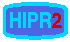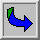# Frequency Domain

For simplicity, assume that the image I being considered is formed by projection from scene S (which might be a two- or three-dimensional scene, etc.).

The frequency domain is a space in which each image value at image position F represents the amount that the intensity values in image I vary over a specific distance related to F. In the frequency domain, changes in image position correspond to changes in the spatial frequency, (or the rate at which image intensity values) are changing in the spatial domain image I.

For example, suppose that there is the value 20 at the point that represents the frequency 0.1 (or 1 period every 10 pixels). This means that in the corresponding spatial domain image I the intensity values vary from dark to light and back to dark over a distance of 10 pixels, and that the contrast between the lightest and darkest is 40 gray levels (2 times 20).

The spatial frequency domain is interesting because: 1) it may make explicit periodic relationships in the spatial domain, and 2) some image processing operators are more efficient or indeed only practical when applied in the frequency domain.

In most cases, the Fourier Transform is used to convert images from the spatial domain into the frequency domain and vice-versa.

A related term used in this context is spatial frequency, which refers to the (inverse of the) periodicity with which the image intensity values change. Image features with high spatial frequency (such as edges) are those that change greatly in intensity over short image distances.